• +91 9971497814
• info@interviewmaterial.com

# RD Chapter 20- Surface Areas and Volume of A Right Circular Cone Ex-20.2 Interview Questions Answers

### Related Subjects

Question 1 :
Find the volume of a right circular cone with:
(i) radius 6 cm, height 7 cm
(ii) radius 3.5 cm, height 12 cm
(iii) height 21 cm and slant height 28 cm. [NCERT]

(i) Radius of a cone (r) = 6 cm
and height (h) = 7 cm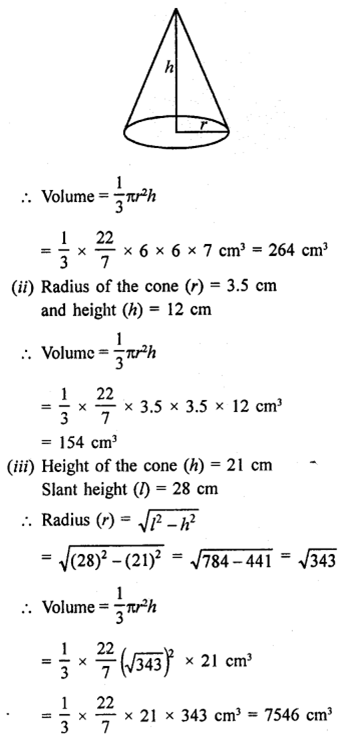Question 2 :
Find the capacity in litres of a conical vessel with:
(i) radius 7 cm, slant height 25 cm,
(ii) height 12 cm, slant height 13 cm. [NCERT]

(i) Radius of the conical vessel (r) = 7 cm
Slant height (h) = 25 cm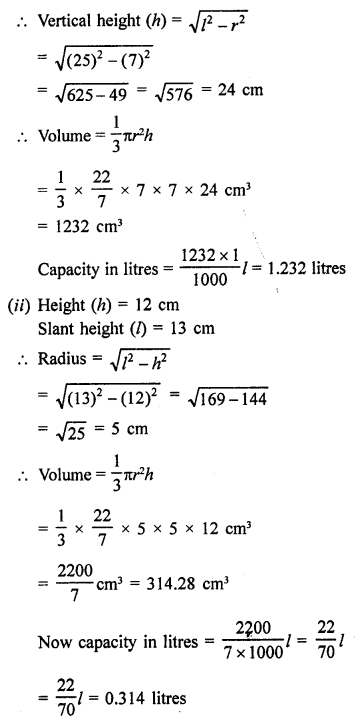Question 3 : Two cones have their heights in the ratio 1 : 3 and the radii of their bases in the ratio 3:1. Find the ratio of their volumes.

Answer 3 : Ratio in the heights of two cones =1:3
and ratio in their radii = 3: 1
Let radius of first cone (r1)= x
and of second cone (r2)= 3x
and height of first cone (h1)= 3y
and of second cone (h2)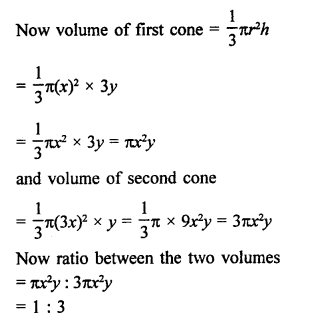Question 4 : The radius and the height of a right circular cone arein the ratio 5 : 12. If its volume is 314 cubic metre, find the slant heightand the radius (Use π = 3.14).

Answer 4 : Ratio in the radius and height of a cone = 5 : 12
Volume = 314 cm3
and height (h) = 12x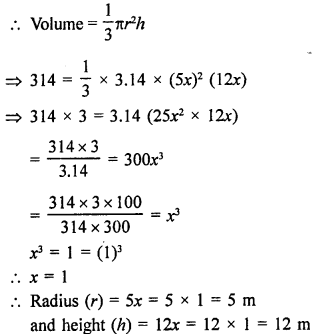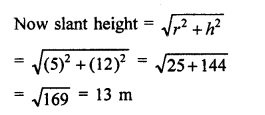Question 5 : The radius and height of a right circular cone are in the ratio 5 : 12 and its volume is 2512 cubic cm. Find the slant height and radius of the cone. (Use π = 3.14).

Answer 5 : Ratio in the radius and height of a right circularcone = 5 : 12
Volume = 2512 cm3
Then height (h) = 12x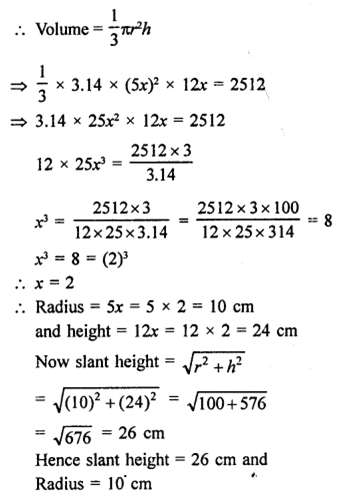Question 6 : The ratio of volumes of two cones is 4 : 5 and the ratio of the radii of their bases is 2:3. Find the ratio of their vertical heights.

Ratio in volumes of two cones = 4:5
and ratio in radii = 2:3
Let radius of the first cone (r1) = 2x
Then radius of second cone (r2) = 3x
Let h1, h2 be their heights respectively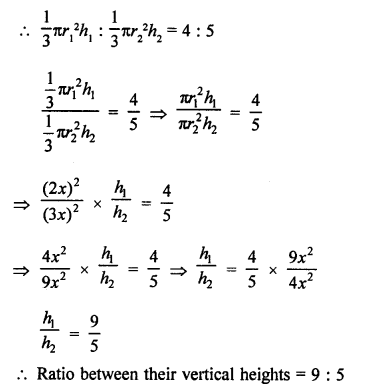Question 7 : Ratio between their vertical heights = 9:5 7. A cylinder and a cone have equal radii of their bases and equal heights. Show that their volumes are in the ratio 3:1.

Answer 7 : Let r be the radius and h be the height of a cylinderand a cone, then
Volume of cylinder = πr2h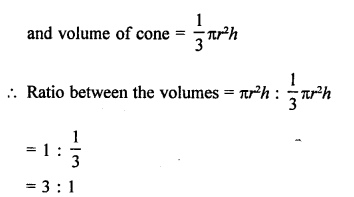Question 8 : If the radius of the base of a cone is halved, keeping the height same, what is the ratio of the volume of the reduced cone to that of the original cone?

Answer 8 : Let r be the radius and h be the height of the cone,then
Volume =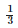πr2h

By halving the radius and same height the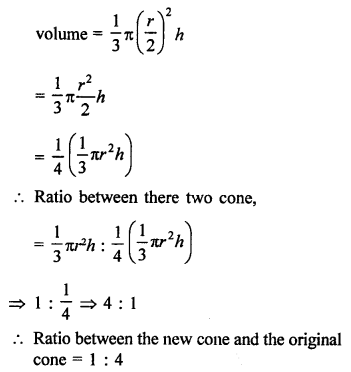Question 9 : A heap of wheat is in the form of a cone of diameter 9 m and height 3.5 m. Find its volume. How much canvas cloth is required to just cover the heap? (Use π = 3.14). [NCERT]

Answer 9 : Diameter of conical heap of wheat = 9 m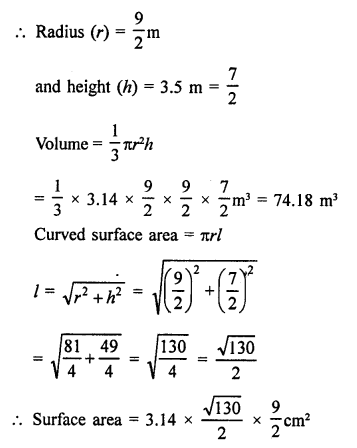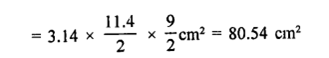Question 10 : Find the weight of a solid cone whose base is of diameter 14 cm and vertical height 51 cm, supposing the material of which it is made weighs 10 grams per cubic cm.

Diameter of the base of solid cone = 14 cm
and vertical height (h) = 51 cm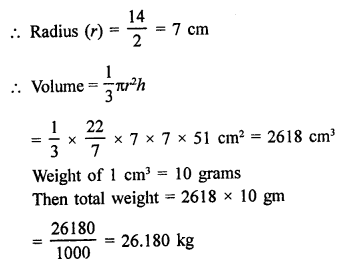Todays Deals### RD Chapter 20- Surface Areas and Volume of A Right Circular Cone Ex-20.2 Contributorskrishan

Name:
Email:

# Latest News# 9000 interview questions in different categories